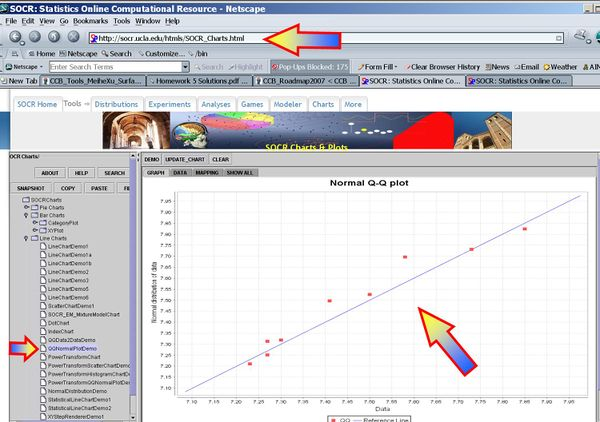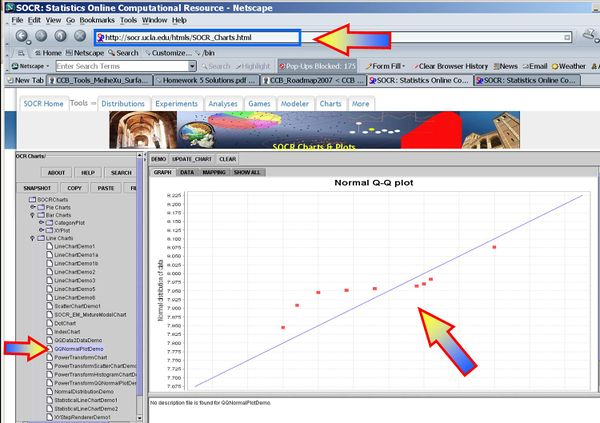# AP Statistics Curriculum 2007 Infer 2Means Indep

## General Advance-Placement (AP) Statistics Curriculum - Inferences about Two Means: Independent Samples

In the previous section we discussed the inference on two paired random samples. Now, we show how to do inference on two independent samples.

### Indepenent Samples Designs

Independent samples designs refer to design of experiments or observations where all measurements are individually independent from each other within their groups and the groups are independent. The groups may be drawn from different populations with different distribution characteristics.

### Background

• Recall that for a random sample {$$X_1, X_2, X_3, \cdots , X_n$$} of the process, the population mean may be estimated by the sample average, $$\overline{X_n}={1\over n}\sum_{i=1}^n{X_i}$$.
• The standard error of $$\overline{x}$$ is given by $${{1\over \sqrt{n}} \sqrt{\sum_{i=1}^n{(x_i-\overline{x})^2\over n-1}}}$$.

### Analysis Protocol for Independent Designs

To study independent samples, we would like to examine the differences between two group means. Suppose {$$X_1, X_2, X_3, \cdots , X_{n_1}$$} and {$$Y_1, Y_2, Y_3, \cdots , Y_{n_2}$$} represent the two independent samples. Then we want to study the differences of the two group means relative to the internal sample variations. If the two samples were drawn from populations that had different centers, then we would expect that the two sample averages will be distinct.

#### Large Samples

• Significance Testing: We have a standard null-hypothesis $$H_o: \mu_X -\mu_Y = \mu_o$$ (e.g., $$\mu_o=0$$). Then the test statistics is:

$Z_o = {\overline{x}-\overline{y}-\mu_o \over SE(\overline{x}-\overline{y})} \sim N(0,1)$. $z_o= {\overline{x}-\overline{y} \over \sqrt{{1\over {n_1}} {\sum_{i=1}^{n_1}{(x_i-\overline{x})^2\over n_1-1}} + {1\over {n_2}} {\sum_{i=1}^{n_2}{(y_i-\overline{y})^2\over n_2-1}}}}$

• Confidence Intervals$(1-\alpha)100%$ confidence interval for $$\mu_1-\mu_2$$ will be

$CI(\alpha): \overline{x}-\overline{y} \pm z_{\alpha\over 2} SE(\overline{x}-\overline{y})= \overline{x}-\overline{y} \pm z_{\alpha\over 2} \sqrt{{1\over {n_1}} {\sum_{i=1}^{n_1}{(x_i-\overline{x})^2\over n_1-1}} + {1\over {n_2}} {\sum_{i=1}^{n_2}{(y_i-\overline{y})^2\over n_2-1}}}$. Note that the $$SE(\overline{x} -\overline{x})=\sqrt{SE(\overline{x})+SE(\overline{y})}$$, as the samples are independent. Also, $$z_{\alpha\over 2}$$ is the critical value for a Standard Normal distribution at $${\alpha\over 2}$$.

#### Small Samples

• Significance Testing: Again, we have a standard null-hypothesis $$H_o: \mu_X -\mu_Y = \mu_o$$ (e.g., $$\mu_o=0$$). Then the test statistics is:

$T_o = {\overline{x}-\overline{y}-\mu_o \over SE(\overline{x}-\overline{y})} \sim T(df)$.

The degrees of freedom is$df={( SE^2(\overline{x})+SE^2(\overline{x}))^2 \over {SE^4(\overline{x}) \over n_1-1} + {SE^4(\overline{y}) \over n_2-1} } \approx n_1+n_2-2.$ Always round down the degrees of freedom to the next smaller integer.

$t_o= {\overline{x}-\overline{y}- \mu_o \over \sqrt{{1\over {n_1}} {\sum_{i=1}^{n_1}{(x_i-\overline{x})^2\over n_1-1}} + {1\over {n_2}} {\sum_{i=1}^{n_2}{(y_i-\overline{y})^2\over n_2-1}}}}$

• Confidence Intervals$(1-\alpha)100%$ confidence interval for $$\mu_1-\mu_2$$ will be

$CI(\alpha): \overline{x}-\overline{y} \pm t_{df, {\alpha\over 2}} SE(\overline{x}-\overline{y})= \overline{x}-\overline{y} \pm t_{df, {\alpha\over 2}} \sqrt{{1\over {n_1}} {\sum_{i=1}^{n_1}{(x_i-\overline{x})^2\over n_1-1}} + {1\over {n_2}} {\sum_{i=1}^{n_2}{(y_i-\overline{y})^2\over n_2-1}}}$. Note that the $$SE(\overline{x} -\overline{x})=\sqrt{SE(\overline{x})+SE(\overline{y})}$$, as the samples are independent.

The degrees of freedom is$df={( SE^2(\overline{x})+SE^2(\overline{x}))^2 \over {SE^4(\overline{x}) \over n_1-1} + {SE^4(\overline{y}) \over n_2-1} } \approx n_1+n_2-2.$ Always round down the degrees of freedom to the next smaller integer. Also, $$t_{df, {\alpha\over 2}}$$ is the critical value for a Student's T distribution at $${\alpha\over 2}$$.

### Example

Nine observations of surface soil pH were made at two different locations. Does the data suggest that the true mean soil pH values differ for the two locations? Formulate testable hypothesis and make inference about the effect of the treatment at $$\alpha=0.05$$. Check any necessary assumptions for the validity of your test.

#### Data in row format

 Location 1 8.1,7.89,8,7.85,8.01,7.82,7.99,7.8,7.93 Location 2 7.85,7.3,7.73,7.27,7.58,7.27,7.5,7.23,7.41

#### Data in column format

Index Location 1 Location 2
1 8.10 7.85
2 7.89 7.30
3 8.00 7.73
4 7.85 7.27
5 8.01 7.58
6 7.82 7.27
7 7.99 7.50
8 7.80 7.23
9 7.93 7.41
Mean 7.9322 7.4600
SD 0.1005 0.2220

#### Exploratory Data Analysis

We begin first by exploring the data visually using various SOCR EDA Tools.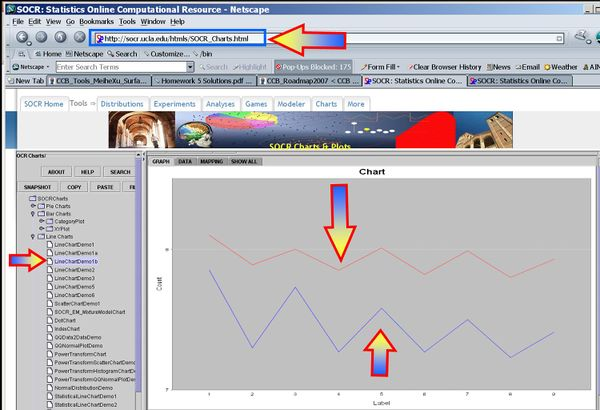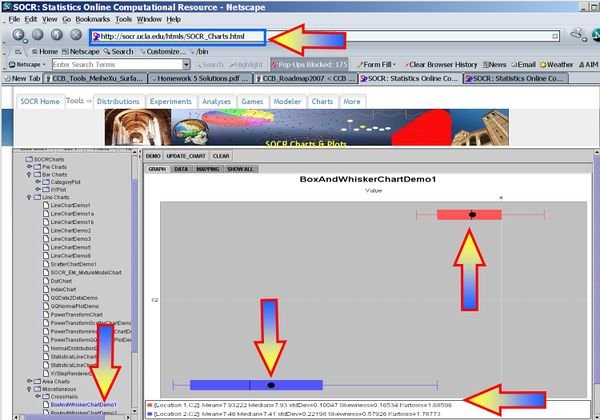#### Inference

• Null Hypothesis$H_o: \mu_{1}-\mu_{2}=0$
• (Two-sided) alternative Research Hypotheses$H_1: \mu_{1} -\mu_{2} \not= 0$.
• Test statistics: We can use the sample summary statistics to compute the degrees of freedom and the T-statistic
The degrees of freedom is$df={( SE^2(\overline{x})+SE^2(\overline{x}))^2 \over {SE^4(\overline{x}) \over n_1-1} + {SE^4(\overline{y}) \over n_2-1} } ={( 0.0335^2+0.074^2)^2 \over {0.0335^4 \over 8} + {0.074^4 \over 8} } = 11.03.$ So, we round down df=11.

$t_o= {\overline{x}-\overline{y}- \mu_o \over \sqrt{{1\over {n_1}} {\sum_{i=1}^{n_1}{(x_i-\overline{x})^2\over n_1-1}} + {1\over {n_2}} {\sum_{i=1}^{n_2}{(y_i-\overline{y})^2\over n_2-1}}}}$

$t_o = {7.9322-7.460-0 \over 0.081}=58.27$.

$p-value=P(T_{(df=11)}>T_o=5.827)=0.00003$ for this (two-sided) test. Therefore, we can reject the null hypothesis at $$\alpha=0.05$$! The left white area at the tails of the T(df=11) distribution depict graphically the probability of interest, which represents the strenght of the evidence (in the data) against the Null hypothesis. In this case, this area is 0.00003, which is much smaller than the initially set Type I error $$\alpha = 0.05$$ and we reject the null hypothesis.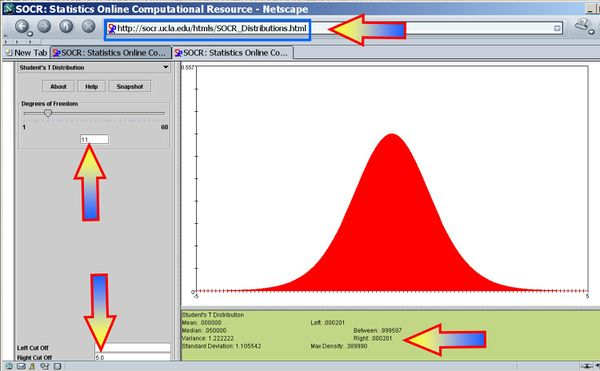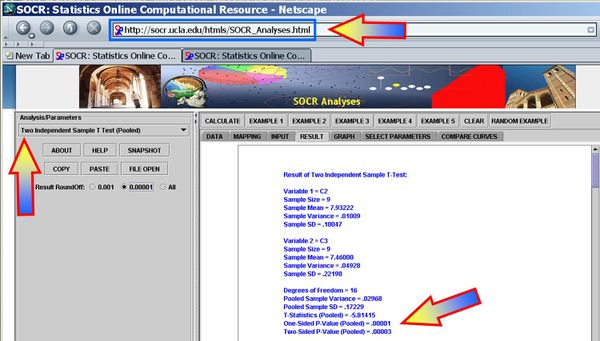• $$95%=(1-0.05)100%$$ ($$\alpha=0.05$$) Confidence interval:

$CI(\mu_{1}-\mu_{2})$$\overline{x}-\overline{y} \pm t_{df, {\alpha\over 2}} SE(\overline{x}-\overline{y})= \overline{x}-\overline{y} \pm t_{df, {\alpha\over 2}} \sqrt{{1\over {n_1}} {\sum_{i=1}^{n_1}{(x_i-\overline{x})^2\over n_1-1}} + {1\over {n_2}} {\sum_{i=1}^{n_2}{(y_i-\overline{y})^2\over n_2-1}}}$

$CI: {7.932-7.460 \pm 2.201\times 0.081 }= [0.294 ; 0.650].$

#### Conclusion

These data show that there is a statistically significant mean difference in the pH of Location 1 and Location 2 (p < 0.001).

#### Independent T-test Validity

Both the confidence intervals and the hypothesis testing methods in the independent-sample design require Normality of both samples. If the sample sizes are large (say >50), Normality is not as critical, as the CLT implies the sampling disitributions of the means are approximately Normal. If these parametric assumptions are invalid we must use a not-parametric (distribution free test), even if the latter is less powerful.

The plots below indicate that Normal assumptions are not unreasonable for these data, and hence we may be justified in using the two independent sample T-test in this case.